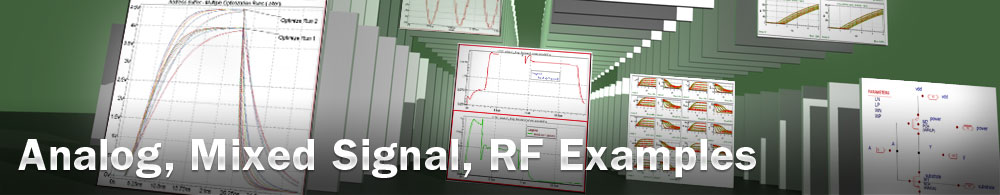MVSG-HV Symmetric GaN HEMT Model Extraction

## opt_ex18 : MVSG-HV Symmetric GaN HEMT Model Extraction

Requires: Utmost IV, SmartSpice, SmartView

Minimum Versions: Utmost IV 2.4.0.R, SmartSpice 4.32.0.R, SmartView 2.34.0.R.

This example describes how to extract an MVSG model for symmetric HEMT devices. To extract a model which is scalable with geometry, multiple different device sizes must be included. In this example, three devices with different gate lengths are included.

The project file opt_ex18.prj and the data file opt_ex18.uds for this example should be loaded into your database. When opened, the project will look as shown in .

Preliminary information

The MVSG model allows independent parameters to describe the source and drain region resistances. In order to consider symmetric devices, with the same parameter values for drain and source, respectively, one can assign two model parameters to the same netlist parameter and optimize the netlist parameter instead: and .

This example uses depletion mode HEMT devices. In order to better display the ID-VG plots of such devices, one needs to edit the preferences: and set the X Axis Direction to "By Sweep" in the Viewer preferences: .

The optimization sequence, which automates the parameter extraction, is divided into ten sections. The objective of each section is to isolate a device characteristic and then to optimize only those model parameters which account for this device behavior. Some model parameters are optimized in multiple sections for better fitting.

Before starting the optimization sequence, the following device parameters are defined in the model library.

• cg: Specific gate capacitance
• lgd: Drain side access region distance
• lgs: Source side access region distance
• tnom: Nominal (room) temperature

Section 1 : idvglin_large_rt

This section performs a preliminary extraction of the threshold voltage for the wide and long channel device, using the drain current versus gate voltage characteristic in the linear region and at room temperature. The extracted value is stored in the vto model parameter, as shown in .

Section 2 : idvglin_large_rt

This section optimizes the parameters for wide and long channel devices. The data in this section is the drain current versus gate voltage characteristic in the linear region and at room temperature. The following model parameters are extracted.

• mu0 Low-field mobility
• ss Subthreshold slope
• vto Threshold voltage

After this step has been completed, the fit to measured data will be as shown in .

Section 3: idvglin_larray_rt

In this section, the parameters for short channel effect are optimized using devices of multiple lengths and wide channel value. The following model parameters are extracted.

• mu0 Low-field mobility
• vto Threshold voltage
• rsh Drain/source sheet resistance

If needed, additional parameters may be considered for improved accuracy.

• mtheta Mobility reduction parameter with VG

After this step has been completed, the fit to measured data will be as shown in .

Section 4: idvglin_large_t

This section extracts the model parameters for the large geometry drain current versus gate voltage linear temperature measurements. The following parameters are optimized.

• vtzeta Threshold voltage dependence on temperature
• epsilon Mobility dependence on temperature

After this step has been completed, the fit to measured data will be as shown in .

Section 5 : idvglin_larray_t

Now we select multiple length devices at temperature for the linear drain current versus gate voltage characteristics and optimize the following parameters.

• vtzeta Threshold voltage dependence on temperature
• epsilon Mobility dependence on temperature
• rct1 Contact and sheet resistance temperature coefficient

After this step has been completed, the fit to measured data will be as shown in .

Section 6: idvglin_larray_rt

This section is used for refining the value of the subthreshold slope parameter.

• ss Subthreshold slope

After this step has been completed, the fit to measured data will be as shown in .

Section 7 : idvgsat_larray_rt

The first 6 sections concentrated only on the linear regions drain current versus gate voltage for each of the different geometries. This section will use the high VDS subthreshold drain current versus gate voltage data for multiple lengths. The following parameters are optimized.

• delta1 DIBL coefficient

After this step has been completed, the fit to measured data will be as shown in .

Section 8 : idvd_larray_rt

This section uses saturation region drain current versus drain voltage data for multiple lengths. The following parameters are optimized.

• vx0 Source injection velocity
• lambda Channel length modulation parameter
• delta1 DIBL coefficient
• rth Thermal resistance

After this step has been completed, the fit to measured data will be as shown in .

Section 9 : all_rt

This section combines room temperature data from all operation regions, in order to refine the nominal temperature parameters.

• mu0 Low-field mobility
• ss Subthreshold slope
• vto Threshold voltage
• rsh Drain/source sheet resistance
• beta Linear to saturation transition parameter
• vx0 Source injection velocity
• lambda Channel length modulation parameter
• delta1 DIBL coefficient
• rth Thermal resistance

After this step has been completed, the fit to measured data will be as shown in .

Section 10 : all_t

Finally, this section combines all temperature data to refine all previously extracted model parameters, including the thermal resistance.

• mu0 Low-field mobility
• ss Subthreshold slope
• vto Threshold voltage
• rsh Drain/source sheet resistance
• beta Linear to saturation transition parameter
• vx0 Source injection velocity
• lambda Channel length modulation parameter
• delta1 DIBL coefficient
• vtzeta Threshold voltage dependence on temperature
• epsilon Mobility dependence on temperature
• rct1 Contact and sheet resistance temperature coefficient
• rth Thermal resistance

If needed, additional parameters may be considered for an improved temperature fitting accuracy.

• vzeta Vx0 dependence on temperature

After this step has been completed, the fit to measured data will be as shown in .

When complete, the model card and the netlist parameters can be exported into an external model library file as shown in the output file opt_ex18.lib.